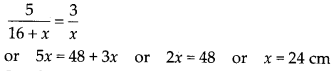Enlightened

# Two charges 5 × 10-88 C and -3 × 10-8 C are located 16 cm apart. At what point(s) on the line joining the two charges is the electric potential zero? Take the potential at infinity to be zero.

• 0

Two charges 5 × 10-88 C and -3 × 10-8 C are located 16 cm apart. At what point(s) on the line joining the two charges is the electric potential zero? Take the potential at infinity to be zero.

Share

1. Solution:
(i) Let C be the point on the line joining the two charges, where electric potential is zero, thenSo, electric potential is zero at distance of 10 cm from charge of 5 × 10-8 C on line joining the two charges between them. If point C is not between the two charges, thenSo, electric potential is also equal to zero a*a distance of 24 cm from charge of -3 × 10-8 C and at a distance of (24 +16) = 40 cm from charge of 5 × 10-8 C, on the side of charge of -3 × 10-8 C.

Check the complete chapter with solutions.

NCERT Solutions for 12th Class Physics: Chapter 2-Electrostatic Potential and Capacitance

• 0